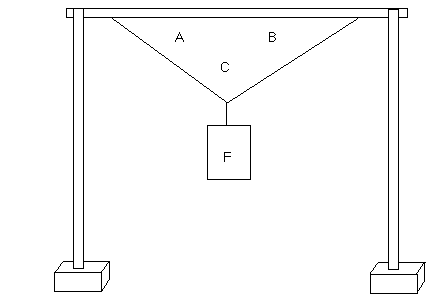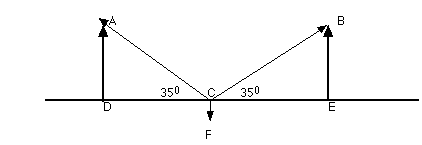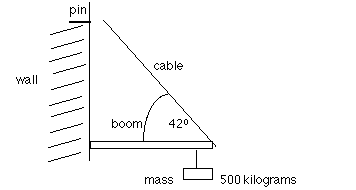## Sunday, 18 December 2005

### Fisika SMA

Pengalaman Belajar Fisika di SMAN BI 1 Banjar

## Experiment: Vector Addition by the Numerical Method

SUBJECT: Mathematics
DESCRIPTION: A set of problems dealing with vector addition.
CONTRIBUTED BY: Carol Hodanbosi
EDITED BY: Jonathan G. Fairman - August 1996

#### Purpose:

A vector is a quantity that has both magnitude, or size, and direction. Forces can be represented by vectors, since they have both a size and direction of action. Below is an example of a mass supported by two cables. If the mass is not moving, all the forces acting on the mass are considered to be balanced. You will investigate each of the forces acting on the mass and compare their relationships.Fw represents the weight of the object. It is found by mulitplying its mass by gravity. That is, Fw = m * g, where g equals 9.8 m/s2. This force is directed downward.

#### Exercises:

1. If the object represented by F has a mass of 4.8 kilograms, find its weight.

2. What direction is the weight of the object acting? Draw this vector through the center of mass of F .

3. The lines CB and CA are vector quantities that are supporting and acting opposite the object F . Draw a line through point C parallel to the base support. Measure the angle between CB and the line just drawn.
Since vectors CB and CA are not acting parallel or perpendicular to the base of the stand, it is helpful to find the components of each of these vectors. Components are vectors that combine vectorally to form the resultant vector, in this case CB or CA . For example, to find the components of CA or CB one first needs to find the angle that vector forms with the horizontal line, angle ACD or angle BCE , see diagram below.To find components of vector CB , form a right triangle with CB as the hypotenuse. Since CB is a vector, or a ray, one will be selecting a fixed portion of CB . Recall the trigonometric functions of the sine (side opposite/ hypotenuse) and the cosine (side adjacent / hypotenuse). The sine of angle BCE would equal side BE/CB , while the sine of angle ACD = AD/ AC .
Let's assume that the angle ACD and angle BCE are both 35° and that the weight represented by vector CF is 100 newtons. Since the weight is static, and not moving, we can assume all the forces are balanced. The vector represented by CE (to the right) must be balanced by the vector CD (to the left).The downward force of the weight represented by CF must be balanced by the two upward forces DA and EB . Since the two right triangles have two congruent angles (35°) and two congruent sides, (FD and CE ) the two triangle are congruent (Leg, Acute angle).
Because DA + EB = CF then DA = EB = 50 newtons.

By substitution, Sin angle BCE = BE/CB

Sin 35° = 50/CB
CB = 50 /Sin 35°
CB = 87.17 newtons
One can also find the measure of CE or CD using the tangent function.
tan BCE = BE/EC
tan 35° = 50 /EC
EC = 50 /tan 35°
EC = 71. 4 newtons

Now use the following diagram to solve the problems below.A load of 500 kg is suspended at the end of a horizontal boom supported by a cable. The cable makes a 42° angle with the boom and is attached to a wall by a supporting pin. You can assume the boom's mass is negligible.
4. Find the downward force vector (the weight of the mass).

5. Represent the components of the cable (one is inward toward the wall, the other is upward, opposite the mass).